# Chapter 16 Models of Yes/No Variables

The techniques studied up until now are intended to model quantitative response variables, those where the value is a number, for example height, wage, swimming times, or foot size. Categorical variables have been used only in the role of explanatory variables.

But sometimes the variation that you want to model occurs in a categorical variable. For instance, you might want to know what dietary factors are related to whether a person develops cancer. The variable “develops cancer” isn’t a number: it’s a yes-or-no matter.

There are good technical reasons why it’s easier to build and interpret models with quantitative response variables. For one, a residual from such a model is simply a number. The size of a typical residual can be described using a variance or a mean square. In contrast, consider a model that tries to predict whether a person will become an businessman or an engineer or a farmer or a lawyer. There are many different ways for the model to be wrong, e.g., it predicts farmer when the outcome is lawyer, or engineer when the outcome is businessman. The “residual” is not a number, so how do you measure how large it is?

This chapter introduces one technique for building and evaluating models where the response variable is categorical. The technique handles a special case, where the categorical variable has only two levels. Generically, these can be called yes/no variables, but the two levels could be anything you like: alive/dead, success/failure, engineer/not-engineer, and so on.

## 16.1 The 0-1 Encoding

The wonderful thing about yes/no variables is that they are always effectively quantitative; they can be naturally encoded as 0 for no and 1 for yes. Given this encoding, you could if you want just use the standard linear modeling approach of quantitative response variables. That’s what I’ll do at first, showing what’s good and what’s bad about this approach before moving on to a better method.

To illustrate using the linear modeling approach, consider some data that relate smoking and mortality. The table below gives a few of the cases from a data frame where women were interviewed about whether they smoked.

Case Outcome Smoker Age
1 Alive Yes 23
2 Alive Yes 18
4 Alive No 67
5 Alive No 64
6 Alive Yes 38

… and so on for 1314 cases altogether.

Of course, all the women were alive when interviewed! The outcome variable records whether each woman was still alive 20 years later, during a follow-up study. For instance, case 3 was 71 years old at the time of the interview. Twenty years later, she was no longer alive.

Outcome is the Yes/No variable I’m interested in understanding; it will be the response variable and smoker and age will be the explanatory variables. I’ll encode “Alive” as 1 and “Dead” as 0, although it would work just as well do to things the other way around.

The simplest model of the outcome is all-cases-the-same: outcome ~ 1. Here is the regression report from this model:

Estimate Std. Error t value p-value
Intercept 0.7192 0.0124 57.99 0.0000

The intercept coefficient in the model outcome ~ 1 has a simple interpretation; it’s the mean of the response variable. Because of the 0-1 coding, the mean is just the fraction of cases where the person was alive. That is, the coefficient from this model is just the probability that a random case drawn from the sample has an outcome of “Alive.”

At first that might seem a waste of modeling software, since you could get exactly the same result by counting the number of “Alive” cases. But notice that there is a bonus to the modeling approach – there is a standard error given that tells how precise is the estimate: 0.719 ± 0.024 with 95% confidence.

The linear modeling approach also works sensibly with an explanatory variable. Here’s the regression report on the model outcome ~ smoker:

Estimate Std. Error t value p-value
(Intercept) 0.6858 0.0166 41.40 0.0000
smokerYes 0.0754 0.0249 3.03 0.0025

You can interpret the coefficients from this model in a simple way – the intercept is group-wise means of outcome for the non-smokers. The other coefficient gives the difference in the means between the smokers and the non-smokers. Since outcome is a 0-1 variable encoding whether the person was alive, the coefficients indicate the proportion of people in each group who were alive at the time of the follow-up study. In other words, it’s reasonable to interpret the intercept as the probability that a non-smokers was alive, and the other coefficient as the difference in probability of being alive between the non-smokers and smokers.

Again, you could have found this result in a simpler way: just count the fraction of smokers and of non-smokers who were alive.

The advantage of the modeling approach is that it produces a standard error for each coefficient and a p-value. The intercept says that 0.686 ±0.033 of non-smokers were alive. The smokerYes coefficient says that an additional 0.075 ±0.05 of smokers were alive.

This result might be surprising, since most people expect that mortality is higher among smokers than non-smokers. But the confidence interval does not include 0 or any negative number. Correspondingly, the p-value in the report is small: the null hypothesis that smoker is unrelated to outcome can be rejected.

Perhaps it’s obvious that a proper model of mortality and smoking should adjust for the age of the person. After all, age is strongly related to mortality and smoking might be related to age. Here’s the regression report from the model outcome ~ smoker+age:

Estimate Std. Error t value p-value
(Intercept) 1.4726 0.0301 48.92 0.0000
smokerYes 0.0105 0.0196 0.54 0.5927
age −0.0162 0.0006 −28.95 0.0000

It seems that the inclusion of age has had the anticipated effect. According to the coefficient −0.016, there is a negative relationship between age and being alive. The effect of smoker has been greatly reduced; the p-value 0.59 is much too large to reject the null hypothesis. These data in fact give little or no evidence that smoking is associated with mortality. (It’s well established that smoking increases mortality, but the number of people involved in this study, combined with the relatively young ages of the smokers, means that the power of the study was small.)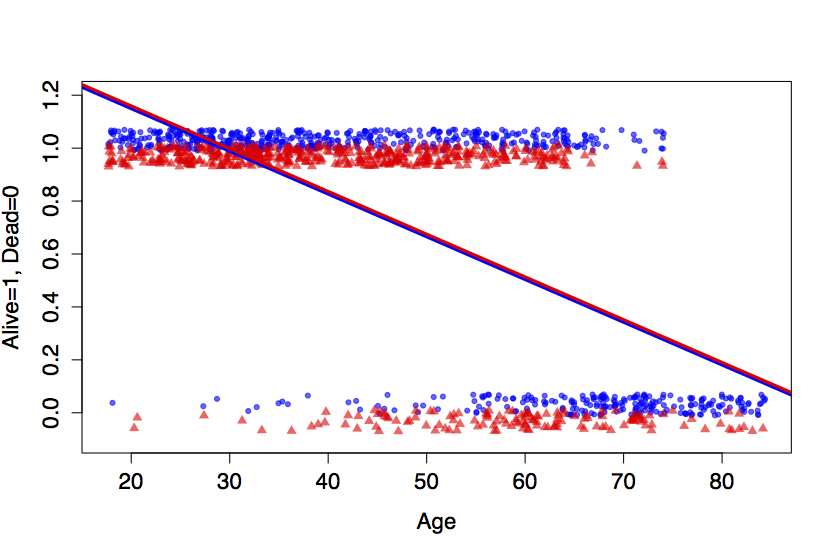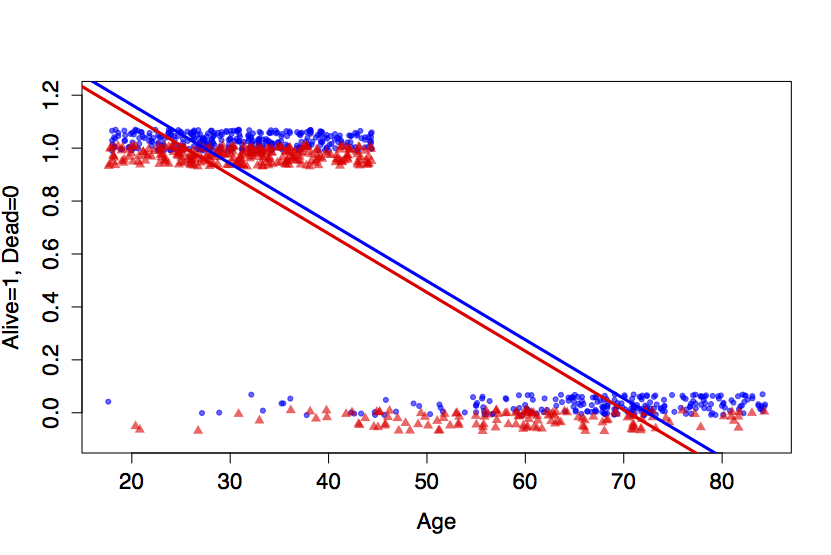Figure 16.1: Top: The smoking/mortality data along with a linear model. Bottom: A thought experiment which eliminates the ‘Alive’ cases above age 45.

There is also a mathematical issue. Consider the fitted model value for a 20-year old smoker: 1.47 + 0.010 - 0.016×0 = 1.16. This value, 1.16, can’t be interpreted as a probability that a 20-year old smoker would still be alive at the time of the follow-up study (when she would have been 40). Probabilities must always be between zero and one.

The top panel of Figure 16.1 shows the fitted model values for the linear model along with the actual data. (Since the outcome variable is coded as 0 or 1, it’s been jittered slightly up and down so that the density of the individual cases shows up better. Smokers are at the bottom of each band, plotted as small triangles.) The data show clearly that older people are more likely to have died. Less obvious in the figure is that the very old people tended not to smoke.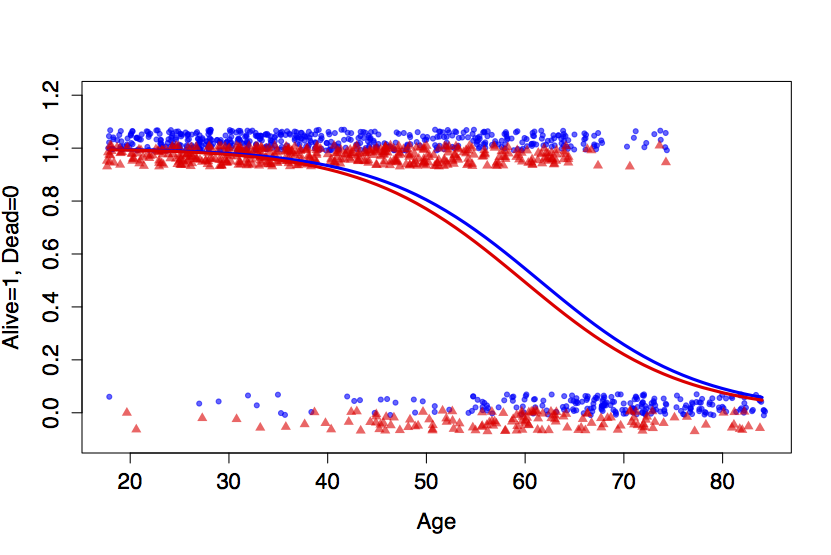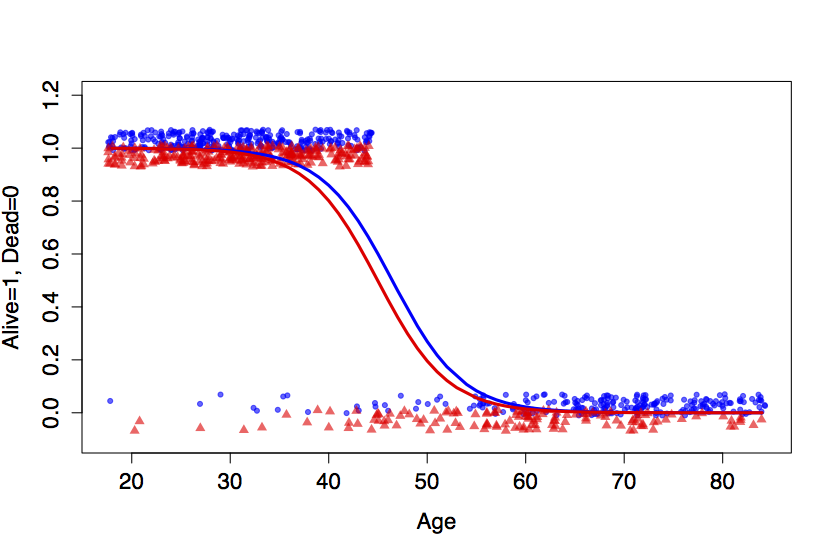Figure 16.2: Logistic forms for outcome ~ age + smoker. Top: The smoking/mortality data. Bottom: The thought experiment in which the ‘Alive’ cases above age 45 were eliminated.

The problem with the linear model is that it is too rigid, too straight. In order for the model to reflect that the large majority of people in their 40s and 50s were alive, the model overstates survival in the 20-year olds. This isn’t actually a problem for the 20-year olds – the model values clearly indicate that they are very likely to be alive. But what isn’t known is the extent to which the line has been pulled down to come close to 1 for the 20-year olds, thereby lowering the model values for the middle-aged folks.

To illustrate how misleading the straight-line model can be, I’ll conduct a small thought experiment and delete all the “alive” cases above the age of 45. The resulting data and the best-fitting linear model are shown in the bottom panel of Figure 16.1.

The thought-experiment model is completely wrong for people in their 50s. Even though there are no cases whatsoever where such people are alive, the model value is around 50%.

What’s needed is a more flexible model – something not quite so rigid as a line, something that can bend to stay in the 0-to-1 bounds of legitimate probabilities. There are many ways that this could be accomplished. The one that is most widely used is exceptionally effective, clever, and perhaps unexpected. It consists of a two-stage process:

1. Construct a model value in the standard linear way – multiplication of coefficients times model vectors. The model outcome ~ age+smoker would involve the familiar terms: an intercept, a vector for age, and the indicator vector for smokerYes. The output of the linear formula – I’ll call it Y – is not a probability but rather a link value: an intermediary of the fitting process.

2. Rather than taking the link value Y as the model value, transform Y into another quantity P that it is bounded by 0 and 1. A number of different transformations could do the job, but the most widely used one is called the logistic transformation:

P = exp(Y) /(1+exp(Y)).

This type of model is called a logistic regression model. The choice of the best coefficients – the model fit – is based not directly on how well the link values Y match the data but on how the probability values P match.

Figure 16.2 illustrates the logistic form on the smoking/mortality data. The logistic transformation lets the model fit the outcomes for the very young and the very old, while maintaining flexibility in the middle to match the data there.

The two-stage approach to logistic regression makes it straightforward to add explanatory terms in a model. Whatever terms there are in a model – main terms, interaction terms, nonlinear terms – the logistic transformation guarantees that the model values P will fall nicely in the 0-to-1 scale. Figure 16.3 shows how the link value Y is translated into the 0-to-1 scale of P for different “shapes” of models.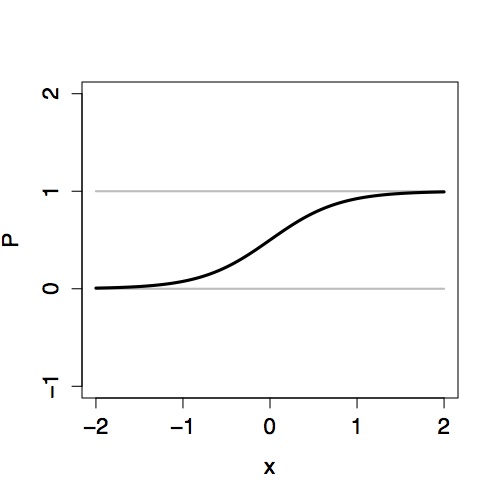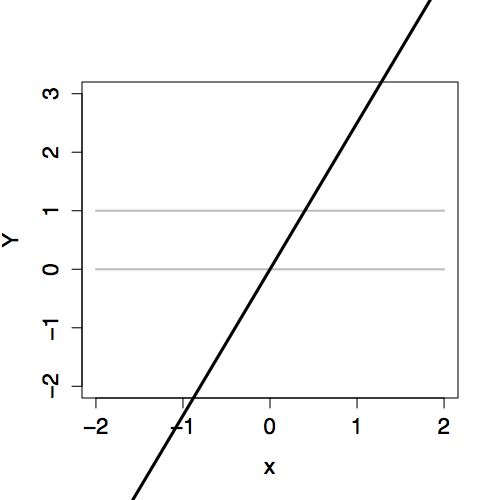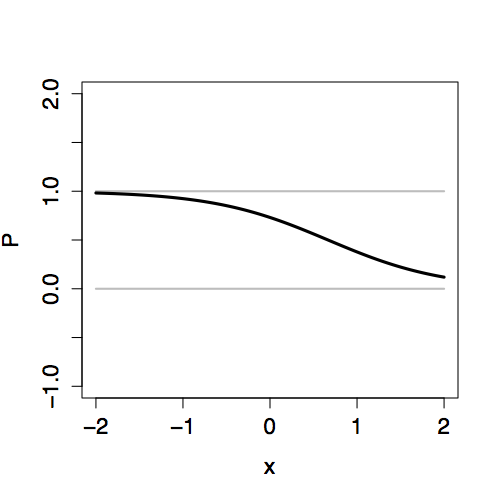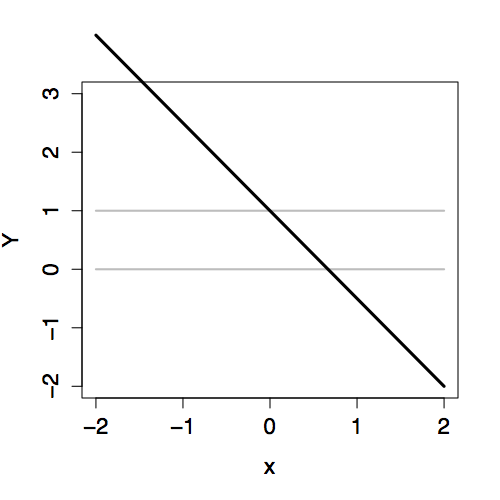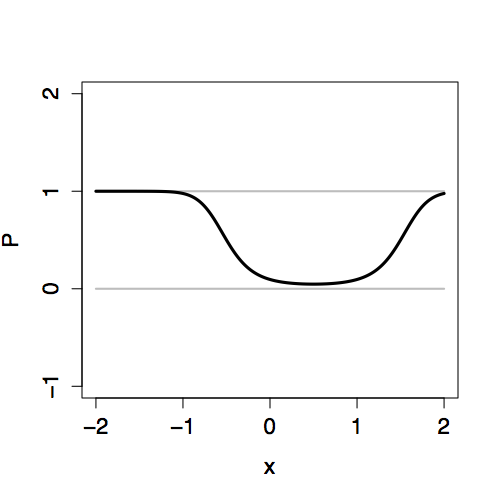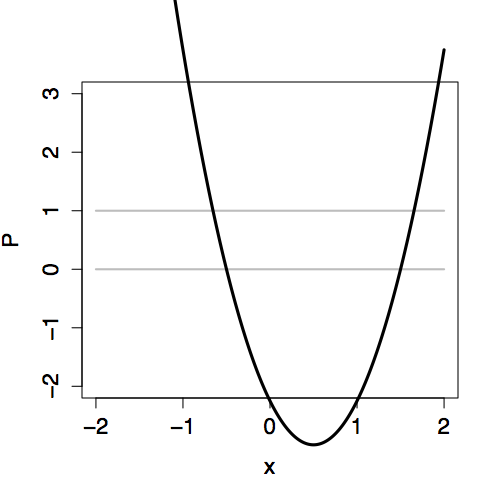Figure 16.3: Comparing the link values Y with the probability values P for three different models, each with an explanatory variable x. Left: Link values. Right: Probability values.

## 16.2 Inference on Logistic Models

The interpretation of logistic models follows the same logic as in linear models. Each case has a model probability value. For example, the model probability value for a 64-year old non-smoker is 0.4215, while for a 64-year old smoker it is 0.3726. These model values are in the form of probabilities; the 64-year old non-smoker had a 42% chance of being alive at the time of the follow-up interview.

There is a regression report for logistic models. Here it is for outcome ~ age+smoker:

Estimate Std. Error z value p-value
Intercept 7.5992 0.4412 17.22 0.0000
age −0.1237 0.0072 −17.23 0.0000
smokerYes −0.2047 0.1684 −1.22 0.2242

The coefficients refer to the link values Y, so for the 64-year old non-smoker the value is Y = 7.59922 - 0.12368 ×64 = -0.3163. This value is not a probability; it’s negative, so how could it be? The model value is the output of applying the logistic transform to Y, that is, exp(-0.3163) /(1+exp(-0.3163)) = 0.4215.

The logistic regression report includes standard errors and p-values, just as in the ordinary linear regression report. Even if there were no regression report, you could generate the information by resampling, using bootstrapping to find confidence intervals and doing hypothesis tests by permutation tests or by resampling the explanatory variable.

In the regression report for the model above, you can see that the null hypothesis that age is unrelated to outcome can be rejected. (That’s no surprise, given the obvious tie between age and mortality.) On the other hand, these data provide only very weak evidence that there is a difference between smokers and non-smokers; the p-value is 0.22.

An ANOVA-type report can be made for logistic regression. This involves the same sort of reasoning as in linear regression: fitting sequences of nested models and examining how the residuals are reduced as more explanatory terms are added to the model.

It’s time to discuss the residuals from logistic regression. Obviously, since there are response values (the 0-1 encoding of the response variable) and there are model values, there must be residuals. The obvious way to define the residuals is as the difference between the response values and the model values, just as is done for linear models. If this were done, the model fit could be chosen as that which minimizes the sum of square residuals.

Although it would be possible to fit a logistic-transform model in this way, it is not the accepted technique. To see the problem with the sum-of-square-residuals approach, think about the process of comparing two candidate models. The table below gives a sketch of two models to be compared during a fitting process. The first model has one set of model values, and the second model has another set of model values.

Model Values
Case 1st Model 2nd Model Observed Value
1 0.8 1.0 1
2 0.1 0.0 1
3 0.9 1.0 1
4 0.5 0.0 0
Sum. Sq. Resid. 1.11 1.00

Which model is better? The sum of square residuals for the first model is (1-0.8)² + (1-0.1)² + (1-0.9)² + (0-0.5)², which works out to be 1.11. For the second model, the sum of square residuals is (1-1.0)² + (1-0.0)² + (1-1.0)² + (0-0.0)², or 1.00. Conclusion: the second model is to be preferred.

However, despite the sum of squares, there is a good reason to prefer the first model. For Case 2, the Second Model gives a model value of 0 – this says that it’s impossible to have an outcome of 1. But, in fact, the observed value was 1; according to the Second Model the impossible has occurred! The First Model has a model value of 0.1 for Case 2, suggesting it is merely unlikely that the outcome could be 1.

The actual criterion used to fit logistic models penalizes heavily – infinitely in fact – candidate models that model as impossible events that can happen. The criterion for fitting is called the likelihood and is defined to be the probability of the observed values according to the probability model of the candidate. When the observed value is 1, the likelihood of the single observation is just the model probability. When the observed value is 0, the likelihood is 1 minus the model probability. The overall likelihood is the product of the individual likelihoods of each case. So, according to the First Model, the likelihood of the observations 1,1,1,0 is 0.8×.1×.9×(1-0.5)= 0.036. According to the Second Model, the likelihood is 1×0×1×(1-0)=0. The First Model wins.

It might seem that the likelihood criterion used in logistic regression is completely different from the sum-of-square-residuals criterion used in linear regression. It turns out that they both fit into a common framework called maximum likelihood estimation. A key insight is that one can define a probability model for describing residuals and, from this, compute the likelihood of the observed data given the model.

In the maximum likelihood framework, the equivalent of the sum of squares of the residuals is a quantity called the deviance. Just as the linear regression report gives the sum of squares of the residual, the logistic regression report gives the deviance. To illustrate, here is the deviance part of the logistic regression report for the outcome ~ age+smoker model:

    Null deviance: 1560.32  on 1313  degrees of freedom
Residual deviance:  945.02  on 1311  degrees of freedom

The null deviance refers to the simple model outcome ~ 1: it’s analogous to the sum of squares of the residuals from that simple model. The reported degrees of freedom, 1313, is the sample size n minus the number of coefficients m in the model. That’s m=1 because the model outcome ~ 1 has a single coefficient. For the smoker/mortality data in the example, the sample size is n=1314. The line labelled “Residual deviance” reports the deviance from the full model: outcome ~ age+smoker. The full model has three coefficients altogether: the intercept, age, and smokerYes, leaving 1314-3=1311 degrees of freedom in the deviance.

According to the report, the age and smokerYes vectors reduced the deviance from 1560.32 to 945.02. The deviance is constructed in a way so that a random, junky explanatory vector would, on average, consume a proportion of the deviance equal to 1 over the degrees of freedom. Thus, if you constructed the outcome ~ age+smoker + junk, where junk is a random, junky term, you would expect the deviance to be reduced by a fraction 1/1311.

To perform a hypothesis test, compare the actual amount by which a model term reduces the deviance to the amount expected for random terms. This is analogous to the F test, but involves a different probability distribution called the χ² (chi-squared). The end result, as with the F test, is a p-value which can be interpreted in the conventional way, just as you do in linear regression.

## 16.3 Model Probabilities

The link values Y in a logistic regression are ordinary numbers that can range from -∞ to ∞. The logistic transform converts Y to numbers P on the scale 0 to 1, which can be interpreted as probabilities. I’ll call the values P model probabilities to distinguish them from the sorts of model values found in ordinary linear models. The model probabilities describe the chance that the Yes/No response variable takes on the level “Yes.”

To be more precise, P is a conditional probability. That is, it describes the probability of a “Yes” outcome conditioned on the explanatory variables in the model. In other words, the model probabilities are probabilities given the value of the explanatory variables. For example, in the model outcome ~ age + smoker, the link value for a 64-year old non-smoker is Y = -0.3163 corresponding to a model probability P = 0.4215. According to the model, this is the probability that a person who was 64 and a non-smoker was still alive at the time of the follow-up interview. That is to say, the probability is 0.4215 of being alive at the follow-up study conditioned on being age 64 and non-smoking at the time of the original interview. Change the values of the explanatory variables – look at a 65-year old smoker, for instance – and the model probability changes.

There is another set of factors that conditions the model probabilities: the situation that applied to the selection of cases for the data frame. For instance, fitting the model outcome ~ 1 to the smoking/mortality data gives a model probability for “Alive” of P=0.672. It would not be fair to say that this is the probability that a person will still be alive at the time of the follow-up interview. Instead, it is the probability that a person will still be alive given that they were in the sample of data found in the data frame. Only if that sample is representative of a broader population is it fair to treat that probability as applying to that population. If the overall population doesn’t match the sample, the probability from the model fitted to the sample won’t necessarily match the probability of “Alive” in the overall population. Often, the interest is to apply the results of the model to a broad population that is not similar to the sample. This can sometimes be done, but care must be taken.

To illustrate, consider a study done by researchers in Queensland, Australia on the possible link between cancer of the prostate gland and diet. Pan-fried and grilled meats are a source of carcinogenic compounds such as heterocyclic amines and polycyclic aromatic hydrocarbons. Some studies have found a link between eating meats cooked “well-done” and prostate cancer.(Koutros and al. 2008) The Queensland researchers(Minchin 2008) interviewed more than 300 men with prostate cancer to find out how much meat of various types they eat and how they typically have their meat cooked. They also interviewed about 200 men without prostate cancer to serve as controls. They modeled whether or not each man has prostate cancer (variable pcancer) using both age and intensity of meat consumption as the explanatory variables. Then, to quantify the effect of meat consumption, they compared high-intensity eaters to low-intensity eaters; the model probability at the 10th percentile of intensity compared to the model probability at the 90th percentile. For example, for a 60-year old man, the model probabilities are 69.8% for low meat-intensity eaters, and 82.1% for high meat-intensity eaters.

It would be a mistake to interpret these numbers as the probabilities that a 60-year old man will have prostate cancer. The prevalence of prostate cancer is much lower. (According to one source, the prevalence of clinically evident prostate cancer is less than 10% over a lifetime, so many fewer than 10% of 60-year old men have clinically evident prostate cancer.)

The sample was collected in a way that intentionally overstates the prevalence of prostate cancer. More than half of the sample was selected specifically because the person had prostate cancer. Given this, the sample is not representative of the overall population. In order to make a sample that matches the overall population, there would have had to be many more non-cancer controls in the sample.

However, there’s reason to believe that the sample reflects in a reasonably accurate way the diet practices of those men with prostate cancer and also of those men without prostate cancer. As such, the sample can give information that might be relevant to the overall population, namely how meat consumption could be linked to prostate cancer. For example, the model indicates that a high-intensity eater has a higher chance of having prostate cancer than a low-intensity eater. Comparing these probabilities – 82.1% and 69.8% – might give some insight.

There is an effective way to compare the probabilities fitted to the sample so that the results can be generalized to apply to the overall population. It will work so long as the different groups in the sample – here, the men with prostate cancer and the non-cancer controls – are each representative of that same group in the overall population. It’s tempting to compare the probabilities directly as a ratio to say how much high-intensity eating increases the risk of prostate cancer compared to low-intensity eating. This ratio of model probabilities is 0.821/0.698, about 1.19. The interpretation of this is that high-intensity eating increases the risk of cancer by 19%.

Unfortunately, because the presence of prostate cancer in the sample doesn’t match the prevalence in the overall population, the ratio of model probabilities usually will not match that which would be found from a sample that does represent the overall population.

Rather than taking the ratio of model probabilities, it turns out to be better to consider the odds ratio. Even though the sample doesn’t match the overall population, the odds ratio will (so long as the two groups individually accurately reflect the population in each of those groups).

Odds are just another way of talking about probability P. Rather than being a number between 0 and 1, an odds is a number between 0 and ∞. The odds is calculated as P/(1-P). For example, the odds 0.50 / (1-0.50) = 1 corresponds to a probability or P=50 % . Everyday language works well here: the odds are fifty-fifty.

The odds corresponding to the low-intensity eater’s probability of P=69.8% is 0.698 / 0.302 = 2.31. Similarly, the odds corresponding to high-intensity eater’s P=82.1% is 0.821 / 0.179 = 4.60. The odds ratio compares the two odds: 4.60 / 2.31 = 1.99, about two-to-one.

Why use a ratio of odds rather than a simple ratio of probabilities? Because the sample was constructed in a way that doesn’t accurately represent the prevalence of cancer in the population. The ratio of probabilities would reflect the prevalence of cancer in the sample: an artifact of the way the sample was collected. The odds ratio compares each group – cancer and non-cancer – to itself and doesn’t depend on the prevalence in the sample.

## Example: Log-odds ratios of Prostate Cancer

One of the nice features of the logistic transformation is that the link values Y can be used directly to read off the logarithm of odds ratios.

In the prostate-cancer data, the model

pcancer ~ age + intensity

has these coefficients:

Estimate Std. Error z value p-value
Intercept 4.274 0.821 5.203 0.0000
Age −0.057 0.012 −4.894 0.0000
Intensity 0.172 0.058 2.961 0.0031

These coefficients can be used to calculate the link value Y which corresponds to a log odds. For example, in comparing men at 10th percentile of intensity to those at the 90th percentile, you multiply the intensity coefficient by the difference in intensities. The bottom 10th percentile is 0 intensity and the top 10th percentile is an intensity of 4. So the difference in Y score for the two percentiles is 0.1722 ×(4 - 0) = 0.6888. This value, 0.6888, is the log odds ratio. To translate this to an odds ratio, you need to undo the log. That is, calculate exp(0.6888) = 1.99. So, the odds ratio for the risk of prostate cancer in high-intensity eaters versus low-intensity eaters is approximately 2.

Note that the model coefficient on age is negative. This suggests that the risk of prostate cancer goes down as people age. This is wrong biologically as is known from other epidemiological work on prostate cancer, but it does reflect that the sample was constructed rather than randomly collected. Perhaps it’s better to say that it reflects a weakness in the way the sample was constructed: the prostate cancer cases tend to be younger than the non-cancer controls. If greater care had been used in selecting the control cases, they would have matched exactly the age distribution in the cancer cases. Including age in the model is an attempt to adjust for the problems in the sample – the idea is that including age allows the model’s dependence on intensity to be treated as if age were held constant.